### El Gusano de Mexicano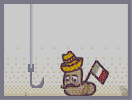Hover over the thumbnail for a full-size version.

Author toby3p0 author:toby3p0 n-art rated 2006-11-30 2006-11-30 4 by 27 people. \$El Gusano de Mexicano#toby3p0#none#000000000000000000000000000000000000000000000000000000000000000000000000000000000000000000000000000000000000000000000000000000000000000000000000000000000Q1OOOH00000000000000000QQ000B0000000000000000000000C00000000000000000000GKM00000000000000000000000000000000000000000000000000000000000000000000000000000000000000000000000000000000000000000000000000000000000000000000000000000000000000000000000000000000000000000000000000000000000000000000000000000000000000000000000000000000000000000000000000000000000000000000000000000000000000000000000000000000000000000000000000000000000000000000000000000000000000000000000000000000000000000000000000000000000000000000000000000000000000000000000000000000000000000000000000000|5^156,564!12^383,398!12^387,399!12^396,399!12^401,399!12^410,399!12^453,383!12^460,376!12^470,369!12^472,366!12^478,361!12^478,361!12^486,356!12^490,350!12^491,350!12^494,354!12^495,366!12^495,372!12^492,381!12^414,432!12^399,432!12^374,422!12^369,414!12^367,403!12^367,397!12^367,396!12^374,398!12^378,398!12^392,399!12^407,399!12^417,398!12^417,398!12^422,398!12^424,397!12^426,394!12^433,391!12^434,390!12^436,390!12^441,388!12^446,384!12^455,379!12^460,374!12^462,372!12^469,368!12^471,365!12^478,360!12^484,354!12^487,352!12^489,351!12^494,351!12^494,360!12^494,369!12^494,370!12^492,382!12^488,393!12^486,397!12^479,406!12^476,409!12^471,415!12^467,418!12^418,432!12^413,432!12^407,432!12^403,432!12^399,432!12^373,417!12^370,412!12^369,406!12^459,422!12^457,422!12^480,404!12^393,397!12^393,388!12^392,382!12^391,382!12^388,373!12^384,362!12^383,361!12^381,356!12^381,351!12^379,343!12^377,337!12^376,333!12^377,330!12^380,330!12^386,329!12^392,329!12^398,329!12^398,328!12^407,322!12^410,318!12^410,314!12^412,310!12^418,309!12^423,309!12^433,318!12^433,318!12^436,322!12^439,328!12^445,335!12^445,335!12^448,343!12^451,345!12^455,350!12^457,356!12^457,360!12^461,365!12^461,367!12^461,369!12^428,311!12^384,365!12^386,368!12^390,377!12^394,395!12^389,364!12^393,364!12^400,364!12^403,364!12^408,364!12^413,362!12^415,359!12^423,354!12^424,352!12^429,349!12^432,347!12^432,346!12^434,344!12^436,343!12^436,343!12^436,342!12^438,340!12^441,336!12^450,350!12^449,351!12^448,354!12^446,358!12^443,362!12^438,364!12^434,366!12^432,368!12^426,373!12^422,373!12^413,378!12^410,379!12^406,380!12^398,380!12^397,380!12^419,375!12^430,369!12^400,380!12^419,355!12^404,326!12^493,377!12^484,400!12^491,387!12^448,425!12^444,426!12^441,426!12^436,427!12^432,428!12^429,429!12^428,429!12^426,430!12^420,431!12^453,423!12^464,419!12^393,431!12^388,429!12^383,427!12^378,425!12^473,411!12^481,357!12^475,363!12^367,394!12^370,390!12^373,387!12^376,384!12^381,381!12^383,378!12^387,379!12^489,348!12^482,348!12^475,348!12^470,348!12^466,349!12^461,350!12^466,372!12^378,408!12^390,408!12^384,402!12^384,414!12^390,420!12^378,420!12^396,426!12^402,420!12^408,414!12^414,408!12^420,402!12^408,402!12^402,408!12^396,414!12^396,402!12^408,426!12^420,426!12^432,426!12^426,420!12^414,420!12^420,414!12^426,408!12^432,402!12^438,396!12^444,390!12^450,396!12^444,402!12^438,408!12^432,414!12^438,420!12^444,414!12^450,408!12^456,402!12^462,408!12^468,414!12^456,414!12^450,420!12^468,402!12^474,396!12^480,390!12^486,384!12^492,378!12^462,396!12^468,390!12^474,384!12^480,378!12^486,372!12^492,366!12^486,360!12^480,366!12^474,372!12^468,378!12^462,384!12^456,390!12^450,384!12^390,342!12^384,336!12^396,336!12^408,336!12^414,330!12^420,324!12^426,318!12^414,318!12^420,312!12^402,342!12^414,342!12^426,342!12^420,336!12^426,330!12^432,324!12^432,336!12^420,348!12^408,348!12^396,348!12^384,348!12^390,354!12^402,354!12^414,354!12^408,360!12^396,360!10^378,390!10^390,390!10^462,354!10^474,354!10^468,360!0^462,360!0^468,354!0^402,336!0^390,336!0^396,342!0^390,348!0^384,342!0^384,354!0^390,360!0^402,360!0^396,354!0^402,348!0^408,342!0^414,336!0^420,330!0^426,324!0^420,318!0^414,324!0^408,330!0^432,330!0^426,336!0^420,342!0^414,348!0^408,354!0^438,336!0^384,390!0^384,384!0^384,408!0^390,414!0^396,420!0^402,426!0^390,426!0^384,420!0^378,414!0^372,408!0^378,402!0^396,408!0^390,402!0^402,414!0^408,420!0^414,426!0^426,426!0^420,420!0^414,414!0^408,408!0^414,402!0^402,402!0^420,408!0^426,414!0^432,420!0^444,420!0^450,414!0^456,408!0^462,402!0^468,396!0^474,390!0^480,384!0^486,378!0^480,372!0^474,378!0^468,384!0^462,390!0^456,396!0^450,402!0^444,408!0^438,414!0^432,408!0^438,402!0^444,396!0^450,390!0^432,396!0^426,402!0^486,366!0^456,384!0^486,390!0^480,396!0^474,402!0^468,408!0^462,414!12^396,384!12^402,390!12^408,396!12^414,390!12^420,384!12^426,378!12^408,384!12^414,378!12^432,384!12^426,390!12^438,378!12^444,372!12^450,366!12^456,360!12^450,354!12^444,360!12^438,366!12^432,372!12^456,372!12^450,378!0^402,396!0^408,390!0^402,384!0^420,390!0^414,384!0^426,384!0^438,384!0^432,378!0^420,378!0^438,372!0^444,378!0^450,372!0^444,366!0^456,366!0^450,360!12^402,400!12^411,400!12^415,400!12^431,395!12^380,399!12^377,399!12^392,383!12^395,388!12^395,392!12^456,354!12^465,361!12^466,364!12^459,361!12^397,377!12^400,375!12^402,371!12^405,368!12^412,369!12^417,371!12^419,366!12^422,361!12^428,363!12^431,363!12^434,359!12^434,356!12^435,351!12^441,351!12^443,351!0^392,370!0^397,370!0^408,372!0^415,366!0^425,367!0^428,355!0^441,358!0^442,344!12^386,432!10^389,442!10^391,440!10^391,440!10^395,438!10^391,442!10^389,446!10^387,449!10^386,453!10^386,457!10^385,465!10^384,469!10^384,474!10^384,474!10^384,487!10^391,550!10^393,555!10^393,558!10^397,559!10^401,562!10^401,563!10^405,563!10^410,567!10^412,570!10^417,570!10^421,574!10^385,478!10^383,481!10^383,486!10^383,504!10^390,554!10^401,563!10^401,564!10^412,571!10^420,574!10^424,574!10^424,574!10^438,574!10^443,574!10^443,574!10^459,574!10^467,574!10^468,574!10^483,574!10^498,573!10^498,573!10^480,411!10^481,413!10^485,413!10^488,417!10^488,418!10^490,426!10^490,429!10^490,438!10^490,445!10^490,447!10^490,458!10^490,467!10^490,475!10^490,481!10^490,488!10^490,495!10^490,503!10^492,507!10^484,410!10^489,415!10^491,424!10^491,430!10^491,438!10^491,438!10^491,445!10^491,453!10^491,462!10^491,468!10^491,475!10^491,485!10^491,493!10^491,493!10^495,501!10^496,504!10^421,575!10^425,576!10^435,576!10^435,576!10^448,576!10^457,576!10^457,576!10^467,575!10^477,575!10^481,575!10^488,575!10^490,575!10^498,571!10^503,571!10^509,571!10^514,571!10^514,571!10^521,570!10^526,570!10^533,570!10^536,569!10^548,566!10^557,562!10^557,561!10^560,557!10^569,547!10^572,540!10^572,532!10^572,521!10^572,516!10^566,510!10^563,506!10^558,504!10^552,499!10^546,497!10^537,496!10^529,495!10^523,495!10^516,496!10^508,496!10^504,494!10^498,491!10^502,491!10^508,496!10^517,497!10^522,497!10^531,497!10^537,497!10^538,497!10^544,497!10^552,497!10^556,502!10^560,509!10^564,513!10^568,515!10^569,522!10^569,528!10^569,539!10^569,542!10^565,552!10^565,552!10^562,555!10^557,559!10^551,563!10^545,567!10^541,567!10^537,568!10^530,569!10^522,570!10^383,490!10^382,493!10^382,496!10^382,500!10^382,501!10^383,506!10^417,512!10^416,510!10^414,509!10^409,507!10^407,507!10^405,507!10^403,510!10^401,512!10^399,515!10^396,518!10^392,522!10^387,523!10^383,523!10^380,523!10^377,523!10^375,523!10^372,519!10^369,517!10^369,520!10^371,524!10^377,528!10^381,528!10^385,528!10^392,528!10^396,524!10^401,520!10^403,517!10^406,512!10^412,512!10^415,514!10^418,510!10^421,509!10^422,508!10^424,505!10^427,505!10^432,505!10^436,507!10^436,507!10^442,509!10^444,514!10^445,515!10^449,516!10^452,517!10^457,517!10^464,517!10^466,517!10^467,515!10^474,512!10^476,511!10^473,512!10^465,516!10^460,517!10^451,518!10^446,515!10^441,511!10^433,506!10^420,515!10^424,515!10^428,515!10^431,515!10^435,515!10^436,516!10^444,522!10^447,523!10^450,523!10^457,523!10^464,522!10^466,519!10^473,515!10^475,513!10^476,511!10^477,510!10^441,523!10^437,520!10^431,518!10^426,518!10^420,519!10^414,521!10^414,521!10^415,522!10^412,520!10^407,520!10^404,522!10^400,523!10^395,527!10^390,530!10^390,544!10^391,549!10^389,545!10^384,509!10^385,509!10^385,507!10^390,546!10^390,546!10^390,547!10^390,547!10^397,460!10^399,458!10^401,456!10^404,456!10^406,455!10^408,454!10^412,451!10^414,451!10^432,450!10^434,450!10^441,450!10^444,450!10^446,451!10^449,452!10^452,452!10^454,453!10^456,454!10^457,454!3^396,467!3^394,473!3^394,480!3^394,485!3^396,491!3^401,493!3^410,493!3^415,486!3^418,478!3^418,472!3^418,465!3^461,458!3^465,463!3^466,472!3^466,479!3^466,488!3^466,494!3^461,496!3^454,496!3^448,496!3^445,493!3^441,488!3^438,485!3^437,478!3^435,474!3^435,467!3^434,461!3^419,458!10^404,481!10^404,479!10^404,479!10^405,479!10^406,480!10^406,482!10^450,482!10^448,479!10^451,479!10^452,480!10^452,483!10^450,484!10^449,480!10^384,391!10^470,356!0^474,355!0^469,357!10^472,354!10^471,355!10^470,357!10^475,511!10^471,515!10^467,517!10^460,517!10^454,517!10^447,517!10^440,512!10^436,507!10^432,504!10^427,504!10^423,504!10^419,506!10^406,510!10^404,511!10^398,516!10^398,517!10^372,523!10^370,521!10^370,520!10^369,518!10^369,517!10^369,517!10^369,516!10^467,519!10^466,519!10^470,517!10^474,512!10^476,508!10^476,508!10^500,569!10^506,569!10^512,569!10^513,569!10^521,570!10^524,570!10^525,570!10^529,570!10^533,568!10^535,568!10^539,568!10^542,566!10^545,565!10^548,564!10^553,562!10^555,559!10^560,555!10^563,553!10^565,551!10^566,549!10^568,547!10^570,543!10^570,541!10^570,537!10^570,534!10^570,532!10^570,530!10^569,524!10^569,522!10^569,522!10^567,515!10^567,515!10^565,511!10^564,509!10^561,506!10^558,504!10^556,503!10^555,501!10^552,498!10^550,497!10^547,497!10^545,497!10^543,497!10^538,497!10^533,497!10^530,497!10^526,497!10^519,497!10^517,497!10^511,495!10^509,495!10^506,493!10^504,491!10^502,491!10^495,505!10^494,505!10^493,502!10^492,501!10^490,494!10^490,490!10^490,486!10^490,485!10^490,479!10^490,472!10^490,469!10^491,464!10^492,462!10^492,462!10^492,458!10^492,456!10^493,454!10^493,450!10^493,446!10^493,443!10^493,441!10^493,437!10^492,433!10^491,430!10^490,428!10^490,423!10^490,421!10^488,419!10^484,415!10^483,414!10^526,570!10^520,570!10^517,570!10^514,570!10^509,572!10^506,572!10^503,574!10^500,574!10^498,574!10^495,575!10^493,575!10^491,575!10^490,575!10^485,575!10^482,575!10^479,575!10^476,575!10^472,575!10^471,575!10^471,575!10^465,575!10^459,575!10^457,575!10^454,575!10^451,575!10^447,575!10^445,575!10^441,575!10^440,575!10^437,575!10^433,575!10^431,577!10^428,577!10^424,575!10^420,572!10^418,571!10^414,570!10^411,569!10^409,568!10^407,566!10^405,564!10^402,562!10^399,561!10^397,559!10^396,557!10^394,555!10^392,550!10^391,548!10^390,546!10^382,497!10^382,492!10^382,490!10^382,487!10^382,481!10^382,479!10^382,476!10^383,473!10^384,473!10^384,470!10^384,464!10^385,461!10^385,461!10^386,455!10^387,452!10^389,447!10^389,446!10^391,443!10^492,367!10^492,371!10^492,378!10^492,381!10^492,385!10^490,389!10^489,392!10^486,397!10^479,405!10^474,409!10^472,410!10^470,411!10^467,413!10^462,417!10^458,418!10^454,419!10^453,419!10^447,424!10^442,425!10^436,426!10^433,427!10^427,428!10^422,430!10^418,431!10^415,431!10^405,432!10^400,432!10^396,432!10^395,432!10^390,432!10^388,432!10^384,429!10^380,425!10^376,422!10^373,418!10^371,414!10^368,410!10^367,405!10^367,401!10^367,397!10^369,390!10^370,386!10^372,383!10^372,383!10^375,383!10^377,382!10^379,382!10^381,380!10^495,365!10^495,358!10^495,352!10^495,351!10^491,349!10^484,347!10^477,347!10^471,347!10^465,347!10^460,347!10^456,349!10^456,351!10^460,356!10^463,359!10^464,362!10^393,391!10^393,389!10^393,387!10^392,383!10^390,380!10^387,375!10^387,374!10^383,367!10^381,361!10^380,358!10^379,353!10^377,348!10^377,342!10^377,338!10^377,334!10^377,333!10^379,328!10^382,328!10^385,328!10^389,329!10^391,331!10^398,331!10^402,325!10^405,321!10^409,314!10^413,311!10^420,310!10^424,310!10^428,315!10^428,319!10^437,322!10^438,323!10^442,328!10^446,333!10^447,336!10^448,341!10^452,342!10^453,343!10^444,333!10^452,353!10^449,355!10^446,356!10^444,359!10^441,361!10^437,362!10^433,363!10^432,366!10^430,368!10^425,370!10^420,373!10^416,376!10^415,376!10^407,376!10^405,377!10^401,377!10^397,377!10^442,334!10^438,337!10^434,342!10^430,345!10^425,347!10^422,350!10^417,353!10^412,355!10^406,358!10^406,358!10^403,360!10^397,361!10^392,361!10^387,361!10^383,361!10^490,351!10^487,354!10^481,358!10^477,362!10^474,365!10^469,369!10^460,376!10^455,379!10^455,379!10^447,383!10^439,385!10^434,388!10^432,390!10^425,391!10^416,394!10^414,394!10^410,394!10^407,394!10^403,394!10^399,395!10^392,396!10^388,396!10^384,396!10^376,395!10^373,394!10^485,415!10^485,417!10^489,420!10^491,420!10^491,425!10^491,427!10^491,435!10^491,444!10^491,448!10^491,452!10^491,463!10^491,468!10^490,476!10^489,485!10^489,489!10^490,494!10^491,500!10^493,504!10^495,504!10^498,489!10^499,491!10^500,493!10^506,493!10^507,493!10^513,493!10^514,498!10^520,498!10^524,498!10^527,498!10^534,498!10^538,498!10^538,498!10^550,498!10^554,502!10^560,506!10^563,508!10^569,513!10^571,519!10^573,526!10^573,535!10^573,540!10^571,546!10^566,551!10^562,559!10^554,563!10^554,563!10^553,564!10^546,568!10^540,569!10^534,570!10^528,571!10^521,571!10^511,571!10^505,572!10^428,576!10^423,576!10^418,574!10^414,571!10^407,568!10^403,565!10^400,560!10^396,556!10^394,553!10^391,551!10^388,547!10^388,544!10^388,542!10^384,499!10^384,505!10^384,509!10^384,512!10^384,484!3^540,552!3^552,540!3^552,552!3^564,528!3^564,540!3^564,516!3^552,528!3^552,516!3^552,504!3^540,504!3^540,516!3^540,528!3^540,540!3^540,564!3^528,564!3^528,552!3^528,540!3^528,528!3^528,516!3^528,504!3^516,504!3^516,516!3^516,528!3^516,540!3^516,552!3^516,564!3^504,564!3^504,552!3^504,540!3^504,528!3^504,516!3^504,504!3^492,504!3^492,516!3^492,528!3^492,540!3^492,552!3^492,564!3^480,564!3^468,564!3^468,552!3^480,552!3^480,540!3^468,540!3^468,528!3^480,528!3^480,516!3^468,516!3^468,504!3^480,504!3^480,492!3^480,480!3^480,468!3^480,456!3^480,444!3^468,456!3^468,444!3^456,444!3^480,432!3^468,432!3^456,432!3^468,420!3^480,420!3^444,432!3^444,444!3^432,444!3^432,432!3^420,444!3^408,444!3^396,444!3^396,456!3^420,432!3^408,432!3^420,456!3^420,468!3^420,480!3^420,492!3^432,492!3^432,480!3^432,468!3^444,504!3^456,504!3^396,504!3^420,528!3^432,528!3^444,528!3^456,528!3^420,528!3^408,528!3^396,528!3^396,540!3^408,540!3^420,540!3^432,540!3^444,540!3^456,540!3^456,552!3^444,552!3^432,552!3^420,552!3^408,552!3^396,552!3^408,564!3^420,564!3^432,564!3^444,564!3^456,564!3^526,508!3^530,510!3^532,517!3^532,520!3^532,526!3^532,529!3^532,534!3^532,548!3^530,550!3^525,557!3^521,561!3^507,502!3^507,509!3^507,521!3^507,528!3^505,535!3^502,541!3^500,545!3^495,556!3^492,561!3^491,562!3^489,565!3^494,555!3^496,546!3^533,510!3^530,505!3^530,508!3^530,510!3^530,514!3^530,520!3^530,525!3^530,525!3^530,533!3^528,537!3^526,542!3^524,546!3^522,551!3^520,554!3^520,555!3^515,562!3^514,562!3^509,508!3^509,512!3^509,519!3^509,526!3^506,533!3^502,538!3^498,548!3^495,554!3^494,555!3^490,561!3^487,563!3^552,536!3^555,523!3^555,547!3^541,547!3^543,515!3^522,520!3^513,537!3^506,553!3^476,554!3^459,558!3^439,559!3^417,557!3^408,545!3^436,544!3^455,544!3^479,542!7^180,396,0!7^180,384,0!7^180,372,0!7^180,360,0!7^180,348,0!7^180,336,0!7^180,324,0!7^180,312,0!7^180,300,0!7^180,288,0!7^180,276,0!7^180,264,0!7^180,252,0!7^180,240,0!7^180,228,0!7^180,216,0!7^180,204,0!7^180,192,0!7^180,180,0!7^180,168,0!7^180,156,0!7^180,144,0!7^180,132,0!7^180,120,0!7^180,108,0!7^180,96,0!7^180,84,0!7^180,72,0!7^180,60,0!7^180,48,0!7^180,36,0!7^180,24,0!7^192,396,2!7^192,384,2!7^192,372,2!7^192,360,2!7^192,348,2!7^192,336,2!7^192,324,2!7^192,312,2!7^192,300,2!7^192,288,2!7^192,276,2!7^192,264,2!7^192,252,2!7^192,240,2!7^192,228,2!7^192,216,2!7^192,204,2!7^192,192,2!7^192,180,2!7^192,168,2!7^192,156,2!7^192,144,2!7^192,132,2!7^192,120,2!7^192,108,2!7^192,96,2!7^192,84,2!7^192,72,2!7^192,60,2!7^192,48,2!7^192,36,2!7^192,24,2!0^474,396!0^450,396!0^456,402!0^432,402!0^402,402!0^390,408!0^414,408!0^438,408!0^462,408!0^474,384!0^468,390!0^474,378!0^480,378!0^432,414!0^408,414!0^384,414!0^402,420!0^426,420!0^420,384!0^444,372!0^414,342!0^390,342!0^420,336!0^402,336!0^396,336!0^426,330!0^408,336!0^396,348!0^552,540!0^552,528!0^552,516!0^540,516!0^540,528!0^540,540!0^540,552!0^552,552!0^528,552!0^528,540!0^528,528!0^528,516!0^516,504!0^516,516!0^516,528!0^516,540!0^516,552!0^504,552!0^492,564!0^480,564!0^480,552!0^492,552!0^504,540!0^504,528!0^504,516!0^504,504!0^492,516!0^492,528!0^492,540!0^480,540!0^480,528!0^468,528!0^468,540!0^468,552!0^468,564!0^456,564!0^444,564!0^432,564!0^432,552!0^444,552!0^456,552!0^456,540!0^444,540!0^432,540!0^432,528!0^420,528!0^420,540!0^420,552!0^408,552!0^408,540!0^396,540!0^408,528!0^444,528!0^456,528!0^480,492!0^480,468!0^480,480!0^480,456!0^480,444!0^480,432!0^468,432!0^468,444!0^456,444!0^456,432!0^444,444!0^432,444!0^420,444!0^408,444!0^444,444!0^444,432!0^420,492!0^432,492!0^396,504!0^456,504!0^468,504!0^408,492!0^426,480!0^426,468!0^426,456!3^552,552!3^540,540!3^528,528!3^516,516!3^504,504!3^540,516!3^552,528!3^528,552!3^516,540!3^504,528!3^492,516!3^504,552!3^492,540!3^480,528!3^480,552!3^492,564!3^468,540!3^456,528!3^468,564!3^456,552!3^444,540!3^432,528!3^444,564!3^432,552!3^420,540!3^408,528!3^408,552!3^396,540!3^480,492!3^480,468!3^480,444!3^456,444!10^516,492!10^516,486!10^522,480!10^522,474!10^528,468!10^528,462!10^534,456!10^534,450!10^540,444!10^540,438!10^546,432!10^546,426!10^552,420!10^552,414!10^558,408!10^558,402!10^564,396!10^564,390!10^570,384!10^570,378!10^576,372!10^576,366!10^582,372!10^588,378!10^594,384!10^600,390!10^606,396!10^612,402!10^618,408!10^624,414!10^630,420!10^636,426!10^642,432!10^648,438!10^648,444!10^642,450!10^642,456!10^636,462!10^636,468!10^630,474!10^630,480!10^624,486!10^624,492!10^618,498!10^618,504!10^612,498!10^606,492!10^600,486!10^594,480!10^588,474!10^582,468!10^576,462!10^570,456!10^564,450!10^558,444!10^552,438!10^517,486!10^518,482!10^519,479!10^520,475!10^525,470!10^527,467!10^528,464!10^528,463!10^529,460!10^530,457!10^532,451!10^535,448!10^536,446!10^538,441!10^542,436!10^543,435!10^544,433!10^545,430!10^546,427!10^546,427!10^546,422!10^550,415!10^551,414!10^552,412!10^553,410!10^554,403!10^558,400!10^558,399!10^564,391!10^568,388!10^570,385!10^572,380!10^573,375!10^577,371!10^579,366!10^575,366!10^574,371!10^572,374!10^569,380!10^568,384!10^565,391!10^563,396!10^560,402!10^546,429!10^542,436!10^538,443!10^534,452!10^530,460!10^523,467!10^521,472!10^519,476!10^516,481!10^514,483!10^584,371!10^588,373!10^589,379!10^593,383!10^594,386!10^599,389!10^601,389!10^602,392!10^603,394!10^606,394!10^611,398!10^613,402!10^615,405!10^617,406!10^622,414!10^624,414!10^630,421!10^632,422!10^638,425!10^639,428!10^639,429!10^641,430!10^645,433!10^646,435!10^646,436!10^646,442!10^646,446!10^646,446!10^644,452!10^642,459!10^641,461!10^639,464!10^635,470!10^632,476!10^629,479!10^627,480!10^626,482!10^624,486!10^623,490!10^622,493!10^619,499!10^618,499!10^614,502!10^611,497!10^610,496!10^604,490!10^603,489!10^597,484!10^597,483!10^594,481!10^591,477!10^586,473!10^584,470!10^580,466!10^577,461!10^573,458!10^570,456!10^569,456!10^566,452!10^563,449!10^560,446!10^558,444!10^556,443!10^552,438!10^551,436!10^549,434!12^618,420!12^618,426!12^612,432!12^612,438!12^606,444!12^606,450!12^600,456!12^600,462!12^594,468!12^594,474!12^600,474!12^606,480!12^612,486!12^612,492!12^618,486!12^612,492!12^606,486!12^600,480!12^618,492!12^618,480!12^624,474!12^630,468!12^630,462!12^636,456!12^636,450!12^642,450!12^642,444!12^642,438!12^636,432!12^630,426!12^624,420!12^624,426!12^630,432!12^624,432!12^618,432!12^618,438!12^624,438!12^630,438!12^636,438!12^636,444!12^630,444!12^624,444!12^618,444!12^612,444!12^612,450!12^618,450!12^624,450!12^630,450!12^630,456!12^624,456!12^618,456!12^612,456!12^606,456!12^606,462!12^612,462!12^618,462!12^624,462!12^624,468!12^618,468!12^612,468!12^606,468!12^600,468!12^606,474!12^612,474!12^618,474!12^606,480!12^612,480!12^624,480!12^617,420!12^616,423!12^614,427!12^613,431!12^612,434!12^611,438!12^609,442!12^608,442!12^605,448!12^602,453!12^598,459!12^598,460!12^596,464!12^602,472!12^605,461!12^610,448!12^614,441!12^615,436!12^620,428!12^625,428!12^630,431!12^632,437!12^639,442!12^636,450!12^628,458!12^625,465!12^621,475!12^610,485!12^610,485!12^607,475!12^608,457!12^615,447!12^620,442!12^626,440!12^631,442!12^626,459!12^616,466!12^615,475!12^612,476!12^609,472!12^611,459!12^618,455!12^624,452!12^628,452!12^631,452!12^634,460!0^570,450!0^576,438!0^582,426!0^588,414!0^594,402!11^762,564,570,444!11^756,564,576,432!11^756,564,582,420!11^750,564,588,408!11^756,564,594,396!0^564,444!0^570,432!0^576,420!0^582,408!0^588,396!0^558,438!0^552,432!0^564,426!0^558,420!0^570,414!0^564,408!0^576,402!0^570,396!0^582,390!0^576,384!11^756,564,564,438!11^750,558,558,432!11^744,558,552,426!11^756,564,570,426!11^756,558,564,420!11^750,558,558,414!11^756,564,576,414!11^756,564,570,408!11^750,558,564,402!11^762,558,582,402!11^744,558,576,396!11^750,552,570,390!11^744,552,588,390!11^744,540,582,384!11^744,552,576,378!0^570,450!0^564,444!0^558,438!0^552,432!0^576,438!0^564,426!0^558,420!0^582,426!0^576,420!0^570,414!0^564,408!0^588,414!0^582,408!0^576,402!0^570,396!0^594,402!0^588,396!0^582,390!0^576,384!0^564,432!0^570,414!0^582,414!11^750,552,564,432!11^750,546,570,420!11^732,528,576,408!11^744,546,582,396!10^515,486!10^511,488!10^518,488!10^515,483!10^521,475!10^524,470!10^526,467!10^526,467!10^527,461!10^530,456!10^531,451!10^533,447!10^537,439!10^540,435!10^543,431!10^543,430!10^550,437!10^552,438!10^557,440!10^558,446!10^559,447!10^561,447!10^567,451!10^570,452!10^571,456!10^571,456!10^578,461!10^581,463!10^581,465!10^585,467!10^585,470!10^587,471!10^595,477!10^597,483!10^599,487!10^606,493!10^608,496!10^610,496!10^614,498!10^616,498!10^621,496!10^622,494!10^623,490!10^626,485!10^627,484!10^628,478!10^628,478!10^634,472!10^637,466!10^639,462!10^641,458!10^644,451!10^646,447!10^646,445!10^646,442!10^646,439!10^645,437!10^642,434!10^639,429!10^634,424!10^630,420!10^627,418!10^622,413!10^617,408!10^613,404!10^611,401!10^608,397!10^606,395!10^603,392!10^602,392!10^602,391!10^600,389!10^598,389!10^596,381!10^594,380!10^590,374!10^586,370!10^584,370!10^576,370!10^576,371!10^574,375!10^572,378!10^566,387!10^562,392!10^559,397!10^556,400!10^554,405!10^552,410!10^550,415!10^548,419!10^546,423!10^546,423!10^543,429!10^537,439!10^535,443!0^36,564!0^60,564!0^84,564!0^108,564!0^132,564!0^156,564!0^180,564!0^204,564!0^228,564!0^252,564!0^276,564!0^300,564!0^324,564!0^348,564!0^372,564!0^372,540!0^348,540!0^324,540!0^300,540!0^276,540!0^252,540!0^228,540!0^204,540!0^180,540!0^156,540!0^132,540!0^108,540!0^84,540!0^60,540!0^36,540!0^36,516!0^60,516!0^84,516!0^108,516!0^132,516!0^156,516!0^180,516!0^276,516!0^300,516!0^324,516!0^348,516!0^372,516!0^48,552!0^72,552!0^96,552!0^120,552!0^144,552!0^168,552!0^192,552!0^216,552!0^240,552!0^264,552!0^288,552!0^312,552!0^336,552!0^360,552!0^564,564!0^588,564!0^612,564!0^636,564!0^660,564!0^684,564!0^708,564!0^732,564!0^756,564!0^756,540!0^732,540!0^708,540!0^684,540!0^660,540!0^636,540!0^612,540!0^588,540!0^588,516!0^612,516!0^636,516!0^660,516!0^684,516!0^708,516!0^732,516!0^756,516!0^36,456!0^84,456!0^132,456!0^180,456!0^228,456!0^276,456!0^324,456!0^372,456!0^516,456!0^564,456!0^660,456!0^708,456!0^756,456!0^576,552!0^600,552!0^624,552!0^648,552!0^672,552!0^696,552!0^720,552!0^744,552!0^24,408!0^72,408!0^120,408!0^168,408!0^216,408!0^264,408!0^312,408!0^360,408!0^504,408!0^552,408!0^648,408!0^696,408!0^744,408!0^48,348!0^144,348!0^288,348!0^528,348!0^624,348!0^720,348!0^24,552!0^768,552!0^768,576!0^744,576!0^720,576!0^696,576!0^672,576!0^648,576!0^624,576!0^600,576!0^576,576!0^552,576!0^384,576!0^408,576!0^360,576!0^336,576!0^312,576!0^288,576!0^264,576!0^240,576!0^216,576!0^192,576!0^168,576!0^144,576!0^120,576!0^96,576!0^72,576!0^48,576!0^24,576!0^756,576!0^732,576!0^708,576!0^684,576!0^660,576!0^636,576!0^612,576!0^588,576!0^564,576!0^540,576!0^396,576!0^372,576!0^348,576!0^324,576!0^300,576!0^276,576!0^252,576!0^228,576!0^204,576!0^180,576!0^156,576!0^132,576!0^108,576!0^84,576!0^60,576!0^36,576!0^24,564!0^48,564!0^72,564!0^96,564!0^120,564!0^144,564!0^168,564!0^192,564!0^216,564!0^240,564!0^264,564!0^288,564!0^312,564!0^336,564!0^360,564!0^384,564!0^576,564!0^600,564!0^624,564!0^648,564!0^672,564!0^696,564!0^720,564!0^744,564!0^768,564!0^768,528!0^744,528!0^720,528!0^696,528!0^672,528!0^648,528!0^624,528!0^600,528!0^360,528!0^336,528!0^312,528!0^288,528!0^264,528!0^240,528!0^216,528!0^192,528!0^168,528!0^144,528!0^120,528!0^96,528!0^72,528!0^48,528!0^24,528!0^744,492!0^720,492!0^696,492!0^672,492!0^648,492!0^600,492!0^576,492!0^552,492!0^360,492!0^336,492!0^312,492!0^288,492!0^264,492!0^240,492!0^216,492!0^192,492!0^168,492!0^144,492!0^120,492!0^96,492!0^72,492!0^48,492!0^768,492!0^24,492!0^60,468!0^108,468!0^156,468!0^204,468!0^252,468!0^300,468!0^348,468!0^540,468!0^684,468!0^732,468!0^732,432!0^768,432!0^696,432!0^660,432!0^528,432!0^360,432!0^324,432!0^288,432!0^252,432!0^216,432!0^180,432!0^144,432!0^108,432!0^72,432!0^36,432!0^216,348!0^96,348!0^348,348!0^576,348!0^672,348!0^672,384!0^624,384!0^768,384!0^720,384!0^768,348!0^528,384!0^348,384!0^288,384!0^216,384!0^144,384!0^96,384!0^48,384!0^24,288!0^108,288!0^180,288!0^252,288!0^324,288!0^408,288!0^492,288!0^552,288!0^636,288!0^708,288!0^768,288# Thank God for math and debate. (where I did almost all of this). Please rate and comment. Just to let you know, the big picture doesn't look too great unless you put it in edit mode. Wow, this was rated up really fast...

## Other maps by this author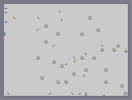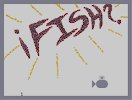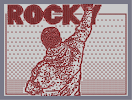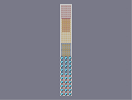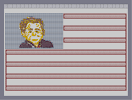Bunch of Laser Drones Físh Rocky Balboa oims One Nation Under Bush

Pages: (0)

### Idiot

It's an Italian flag. French is Red White Blue.

Very Nice 4/5aved

### Pretty cool,

but you could have added something to the upper part of the level. Sorry, but why does he have the French flag? Well, it doesn't matter. I know how hard it is to fill a small area with green color. A good N art nonetheless. You've done your best in a short time. 4/5.

### Hey Jack youre late for the maths debate...

Great art! I like to see ones like this 5/5

### OMG THIS IS HORRIBLE

jk its really good....

### Ahahaha!

Perfect. The reason N art exists. Naved, Where N = 5.

### heh

Math-debating.

good job dude. 4.5/5

### Nice

Very nice... Like the flag

### nice map 5/5

great job....too bad you couldnt make the flag green

### You did all of this whilst math debating?

You must be quick at making these!

### wierd

this is kind of random